Miscellaneous

Chapter 16 Class 11 Probability
Serial order wise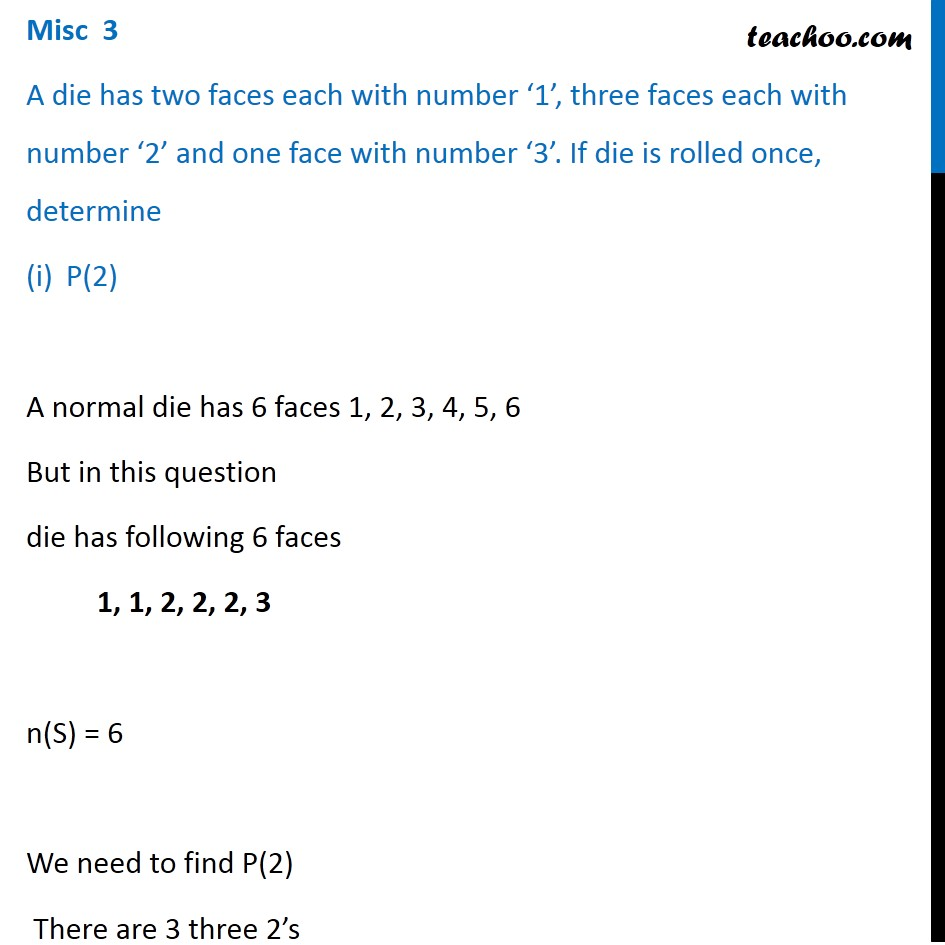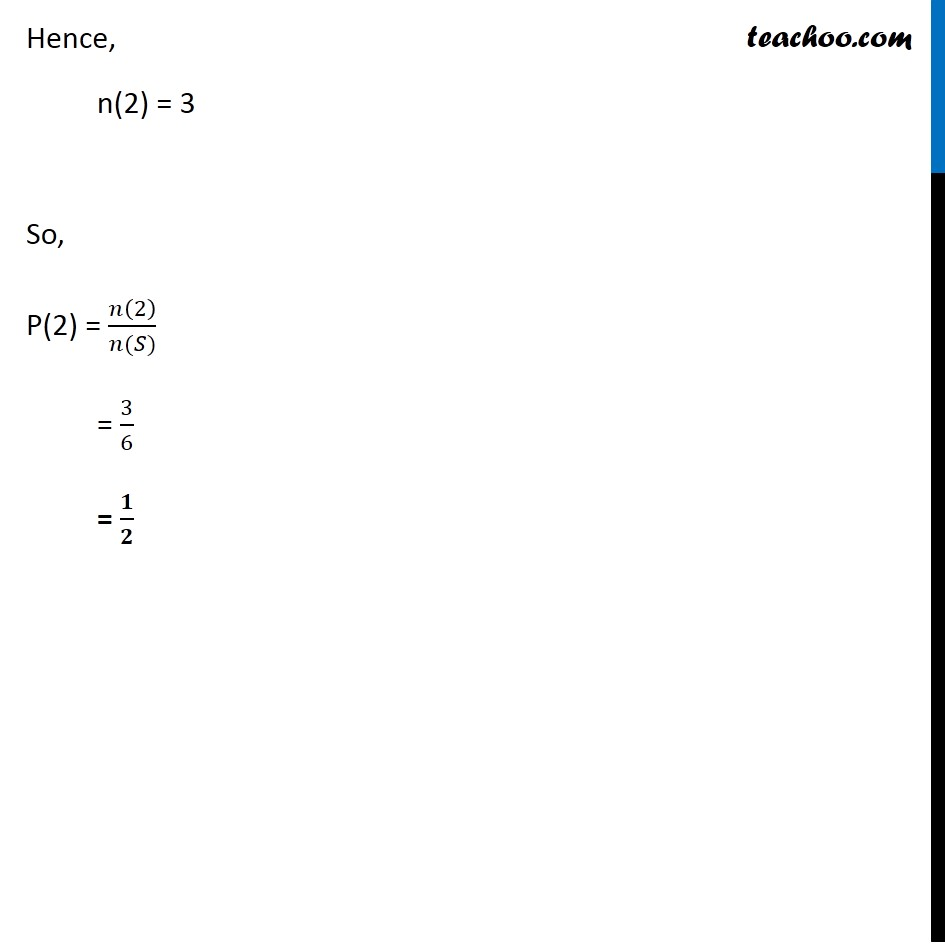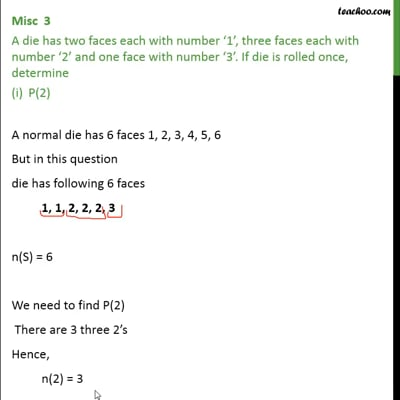This video is only available for Teachoo black users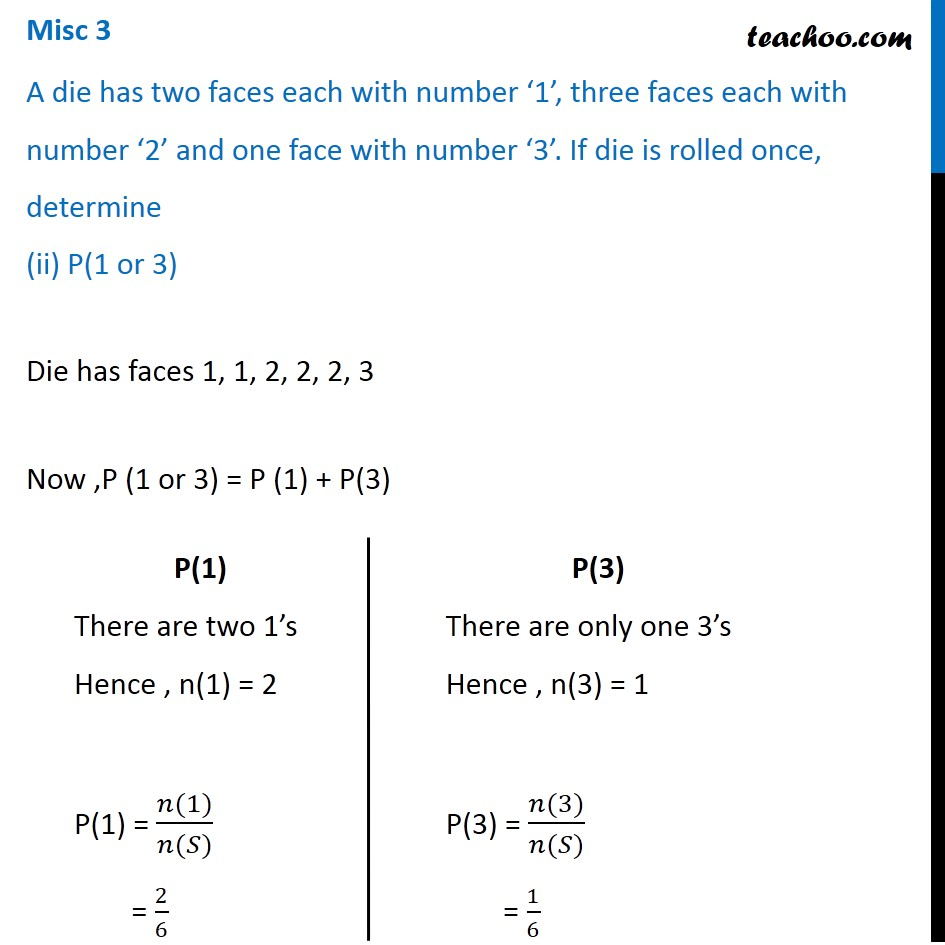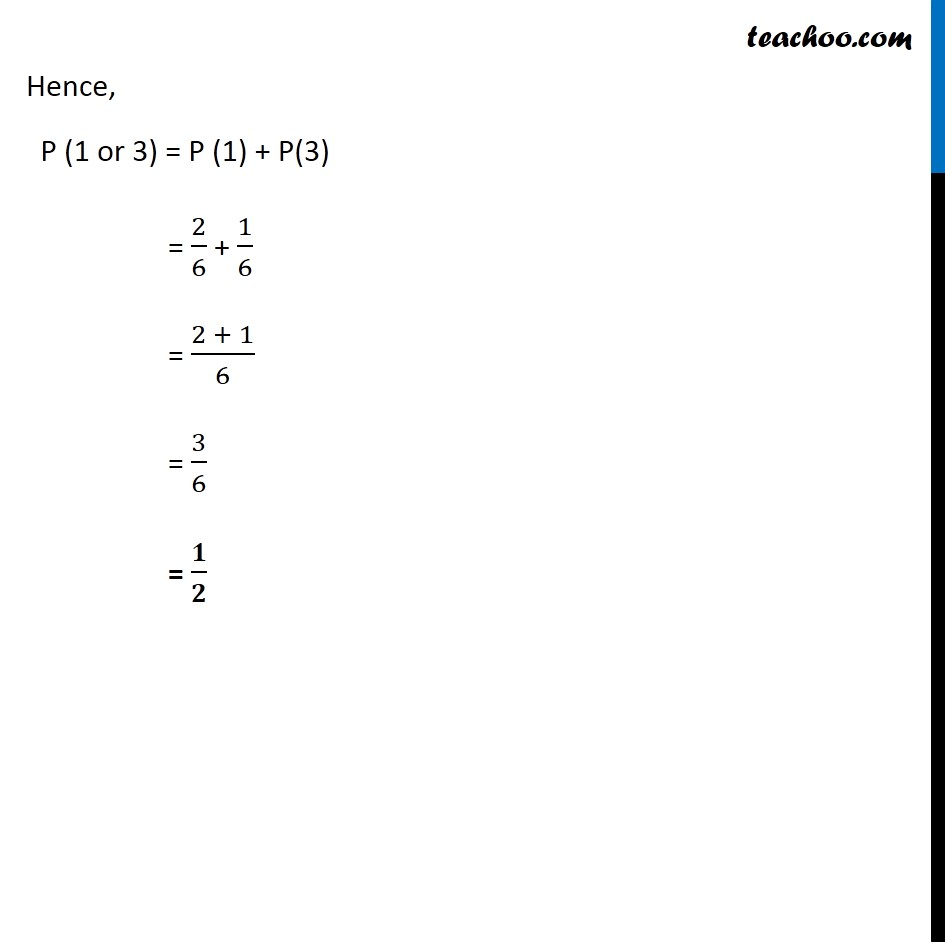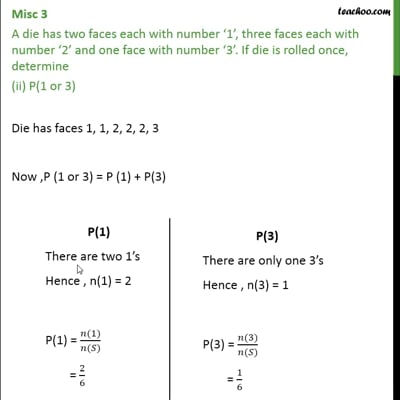This video is only available for Teachoo black users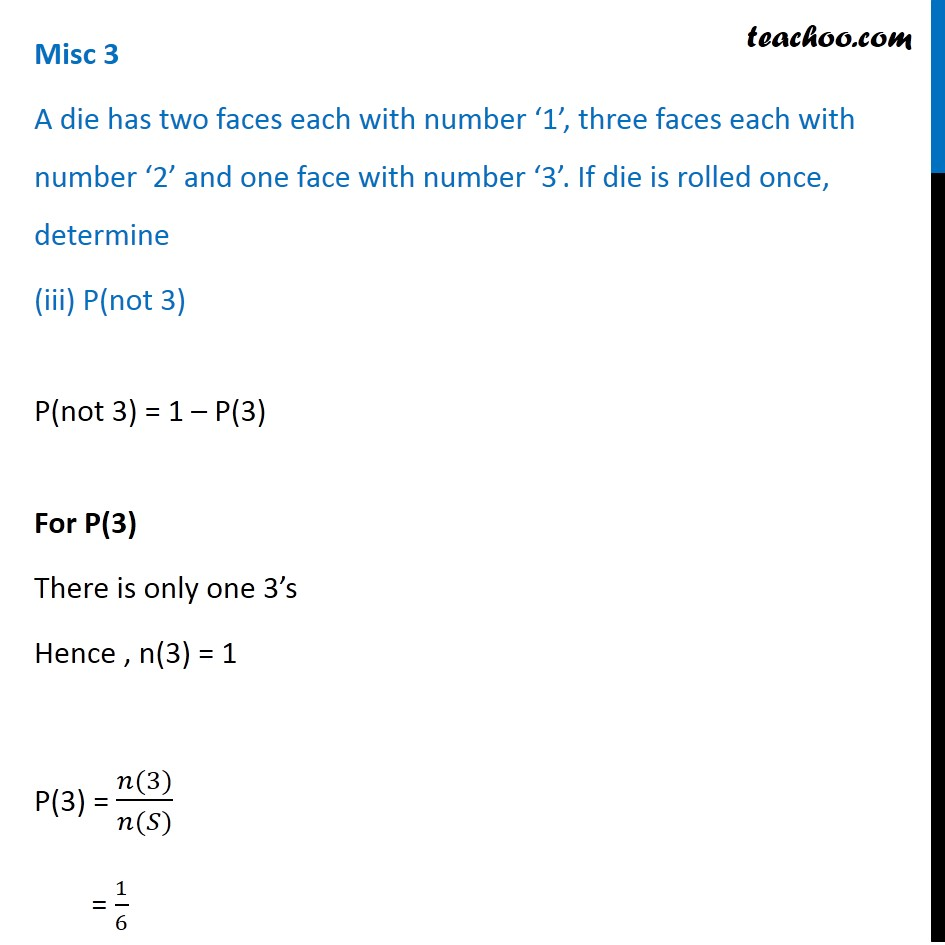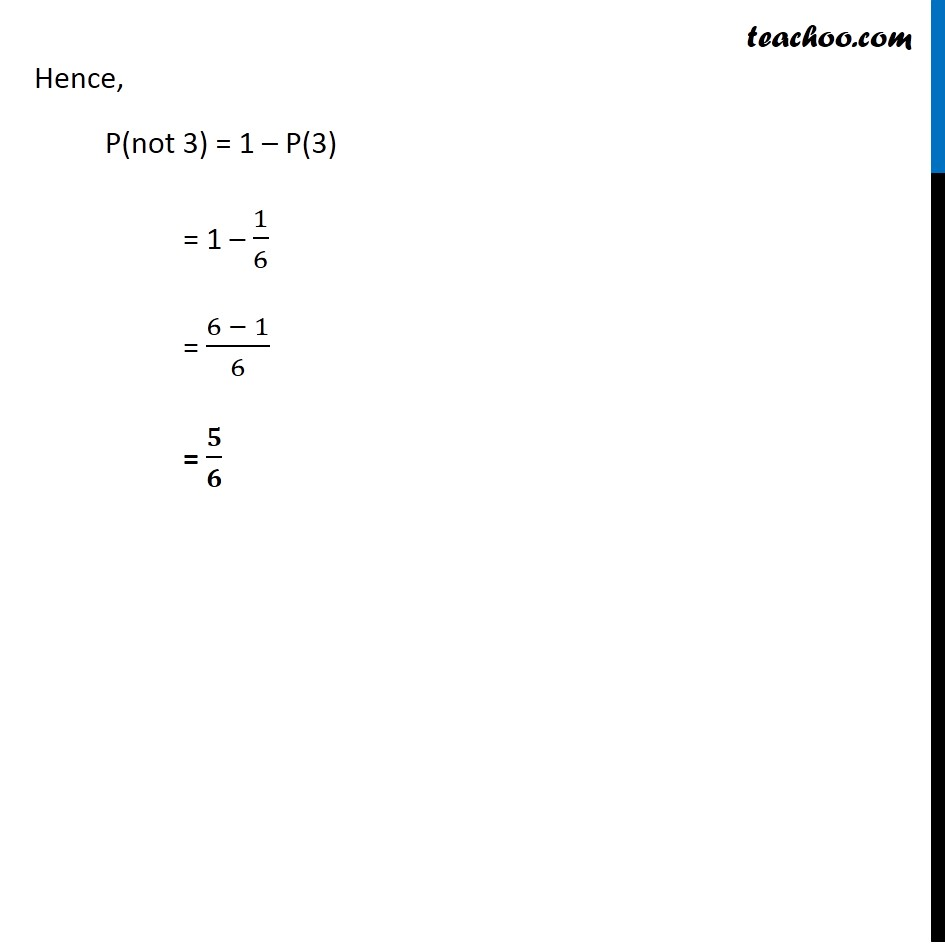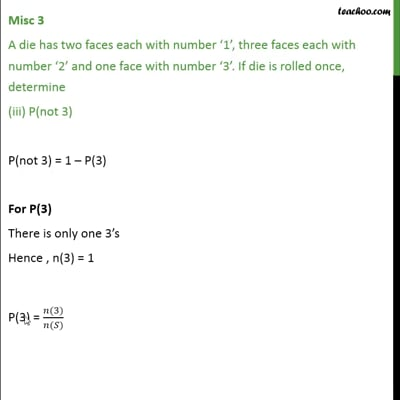This video is only available for Teachoo black users

Solve all your doubts with Teachoo Black (new monthly pack available now!)

### Transcript

Misc 3 A die has two faces each with number ‘1’, three faces each with number ‘2’ and one face with number ‘3’. If die is rolled once, determine P(2) A normal die has 6 faces 1, 2, 3, 4, 5, 6 But in this question die has following 6 faces 1, 1, 2, 2, 2, 3 n(S) = 6 We need to find P(2) There are 3 three 2’s Hence, n(2) = 3 So, P(2) = 𝑛(2)/(𝑛(𝑆)) = 3/6 = 𝟏/𝟐 Misc 3 A die has two faces each with number ‘1’, three faces each with number ‘2’ and one face with number ‘3’. If die is rolled once, determine (ii) P(1 or 3) Die has faces 1, 1, 2, 2, 2, 3 Now ,P (1 or 3) = P (1) + P(3) P(1) There are two 1’s Hence , n(1) = 2 P(1) = (𝑛(1))/(𝑛(𝑆)) = 2/6 P(3) There are only one 3’s Hence , n(3) = 1 P(3) = (𝑛(3))/(𝑛(𝑆)) = 1/6 Hence, P (1 or 3) = P (1) + P(3) = 2/6 + 1/6 = (2 + 1)/6 = 3/6 = 𝟏/𝟐 Misc 3 A die has two faces each with number ‘1’, three faces each with number ‘2’ and one face with number ‘3’. If die is rolled once, determine (iii) P(not 3) P(not 3) = 1 – P(3) For P(3) There is only one 3’s Hence , n(3) = 1 P(3) = (𝑛(3))/(𝑛(𝑆)) = 1/6 Hence, P(not 3) = 1 – P(3) = 1 – 1/6 = (6 − 1)/6 = 𝟓/𝟔